Want to share your content on R-bloggers? click here if you have a blog, or here if you don't.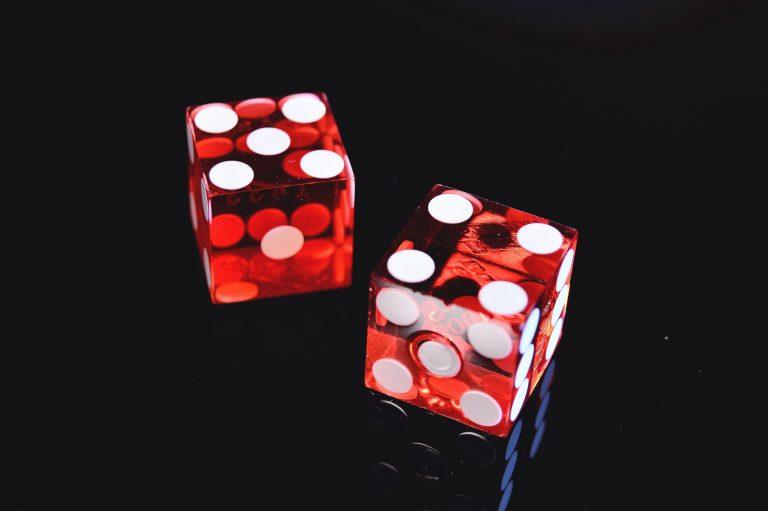In my last post I coded Liar’s Dice in R and some brainless bots to play against. I build on that post by using Q-learning to train an agent to play Liar’s Dice well.

Spoiler alert: The brainless bots aren’t actually that brainless! More on that later.

Note – The full code is too long to share here so you can find it on Github. I’ll only be sharing the key bits and simulation results. Check out my previous post for the rules to Liar’s Dice.

## What is Q-learning?

Firstly, some background. Q-learning is a reinforcement learning algorithm which trains an agent to make the right decisions given the environment it is in and what tasks it needs to complete. The task may be navigating a maze, playing a game, driving a car, flying a drone or learning which offers to make to increase customer retention. In the case of Liar’s Dice, when to call or raise the bid. The agent learns through

• Exploring the environment
• Choosing an action
• Receiving a penalty or reward and
• Storing that information to do better the next time.

This cycle is repeated until eventually it learns the best decisions to make given it’s situation.

The environment is formulated as a Markov Decision Process defined by a set of states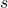. By taking an actionthe agent will transition to a new statewith probability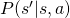. After transitioning to the new state the agent receives a rewardwhich will either tell it this was a good move, or this was a bad move. It may also tell the agent this was neither a good or bad move until it reaches a win/lose state.

Finding the optimal policy of an MDP can be done through value iteration and policy iteration. The optimal policy and state value functions are given by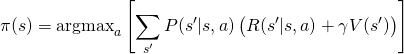and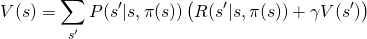where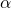and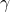are learning rate and discount parameters. The above equations rely on knowing (or often, making crude approximations to) the transition probabilities. The benefit of Q-learning is the transition probabilities are not required, instead they are derived through simulation. The Q function is given by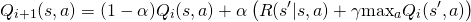The cells in the Q matrix represent the ‘quality’ of taking actiongiven  state. After each action the Q matrix is updated. After many iterations, the agent would have explored many states and determined which states and action pairs led to the best outcomes. Now it has the information needed to make the optimal choice by taking the action which leads to the maximum overall reward indicated by the largest Q value.

## Liar’s Dice Markov Decision Process

### State space

The key is to formulate the states the agent can be in at any point in the game and the reward for transitioning from one state to another. MDP’s can become very large very quickly if every possible state is accounted for so it’s important to identify the key information and the redundancies.

The key pieces of information needed to make a decision in Liar’s Dice are

• The total number of dice on the table
• The number of dice in the players possession
• The players roll of the dice and
• The bid

Consider the player has 6 dice, this gives a possible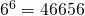possible hands and this hasn’t yet factored in the bid or the total number of dice on the table. You can see how the number of states blows out.

To make a good decision on whether or not to raise or call the player only needs to know how many dice of the current bid value the player has in their hand and the chance the remainder are in the unseen opponents dice. Essentially, the dice value isn’t required in the formulation of the game state.

The states are given by 3 values.

• The total number of dice on the table
• The number of dice in the players possession
• The probability bucket e.g. 10%, 20%, etc

The last point is the combination of the information given by the players dice and the bid. The probability there is at least the bid quantity on the table is calculated and reduced to a bucket.whereand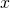is the unknown quantity needed and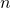is the number of unobserved dice on the table. This reduces the state space down to something more manageable. For this example we’ll use a maximum of 20 buckets i.e. (5%, 10%, …, 100%). Overkill for small numbers of dice, but it doesn’t hurt.

The function below generates the complete game states given the number of dice and players.

# generate games states
generate.game.states <- function(nplayers, ndice, bin.size = 20){

# create the basic data frame with total dice and player dice count
total.dice <- nplayers*ndice
states <- t(combn(rep(0:ndice, nplayers), nplayers)) %>% as.data.frame() %>% distinct()
colnames(states) <- paste0("p", 1:nplayers)
states$total <- rowSums(states) states <- states %>% dplyr::select(total, p1) %>% dplyr::arrange(total, p1) %>% dplyr::filter(total > 0) %>% distinct # add in the probability bucket state game.states <- data.frame() for(k in 1:nrow(states)){ total <- states$total[k]
p1 <- game.states$p1[i] # small penalty for losing a die reward[i, p1 - game.states$p1 == 1 & total - game.states$total == 1 & game.states$p1 != game.states$total] <- -1 # small reward for others losing a die reward[i, p1 == game.states$p1 & total - game.states$total == 1 & game.states$p1 != game.states$total & p1 > 0] <- 1 # fail states - when players dice count is 0 if(p1 == 1){ reward[i, which(total - game.states$total == 1 & game.states$p1 == 0)] <- -10 } # win states when the player dice count equals the total dice count if(total - p1 == 1){ reward[i, game.states$total == p1 & game.states$p1 == p1] <- 10 } } return(reward) } rw <- generate.reward.matrix(gs) reward <- list(raise = rw, call = rw) ## The process The process follows the steps below.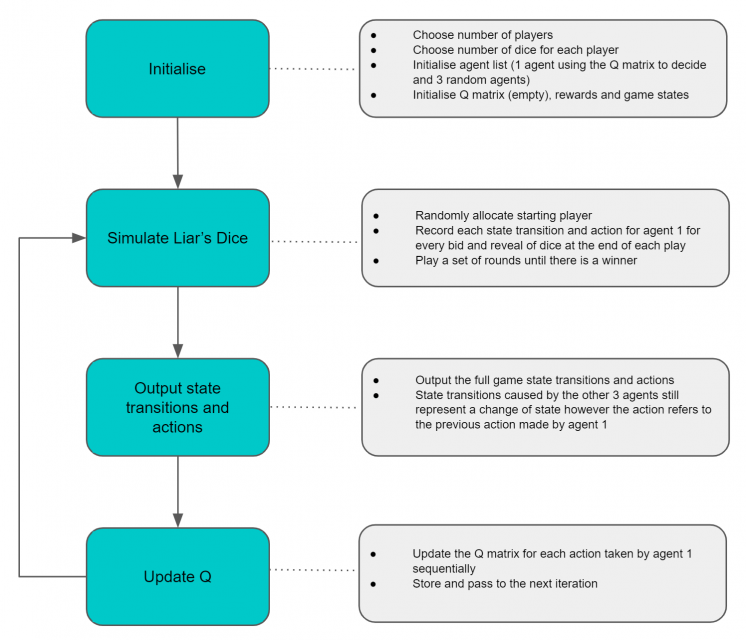Assume player 1 raises on their turn. In a 4 person game, player 1 may actually transition to multiple other states before control returns. For each other raise or call by the other players, the game state will change for player 1. For the context of the model the action player 1 took is considered to be the last action for all subsequent transitions. Here is an example of the state transition table for 4 players each with 3 dice. play.liars.dice(auto = TRUE, players = 4, num.dice = 3, verbose = 0, agents = agents, Q.mat = Q.mat, print.trans = TRUE)$winner

##    y.ctrl y.state y.action
## 1       3     808    raise
## 2       3     787    raise
## 3       3     778    raise
## 4       4     778    raise
## 5       4     736    raise
## 6       4     736    raise
## 7       1     736     call
## 8       1     673     call
## 9       1     678    raise
## 10      2     674    raise
## 11      3     673    raise
## 12      4     673    raise
## 13      4     589    raise
## 14      4     592    raise
## 15      1     589     call
## 16      1     505     call
## 17      1     507     call
## 18      1     423     call
## 19      2     422    raise
## 20      3     421    raise
## 21      1     421    raise
## 22      2     421    raise
## 23      2     316    raise
## 24      2     324    raise
## 25      2     240    raise
## 26      3     232    raise
## 27      3     148    raise
## 28      2     151    raise
## 29      2      88    raise

##  1

This table is then passed to the update.Q() function.

# update Q matrix
update.Q <- function(play, Q.mat, reward, alpha = 0.1, discount = 0.9){
for(k in 2:nrow(play)){
curr.state <- play$y.state[k] prev.state <- play$y.state[k-1]
action <- play$y.action[k] # Q update Q.mat[prev.state, action] <- (1-alpha)*Q.mat[prev.state, action] + alpha*(reward[[action]][prev.state, curr.state] + discount*max(Q.mat[curr.state,])) } return(Q.mat) } The learning rate and discount values have been initialised to 0.1 and 0.9 respectively. ## The simulation Liar’s Dice is now simulated 5000 times and Q value iteration is conducted with the above functions (see github for the full code). The first agent will be the only one that uses the Q matrix to decide it’s actions and therefore the only agent that is trained. It will bluff with a probability of 50% to add in some more realism to the agents decision. The other 3 will be random agents, bluffing 100% of the time and randomly deciding to call or raise at each decision point. It is expected that after training agent 1 will outperform the other 3 random agents. # set the agents agent1 <- build.agent(c(0.5,0.5), method = "Q.decide") agent2 <- build.agent(c(1,0)) agent3 <- build.agent(c(1,0)) agent4 <- build.agent(c(1,0)) agents <- list(agent1, agent2, agent3, agent4) # training its <- 5000 # setting the vector to store the winners winners <- vector("numeric", length = its) # loopy loop pb <- txtProgressBar(min = 0, max = its, style = 3) for(k in 1:its){ out <- play.liars.dice(auto = TRUE, players = 4, num.dice = 6, verbose = 0, agents = agents, Q.mat = Q.mat, train = TRUE) winners[k] <- out$winner
Q.mat <- out\$Q.mat
setTxtProgressBar(pb, k)
}

# table of winners
table(winners)

## winners
##    1    2    3    4
## 3288  434  496  782

# agent win percentage
x <- 1000:5000
y <- (cumsum(winners == 1)/(1:5000))[x]
qplot(x = x, y = y, geom = "line", xlab = "iterations", ylab = "Proportion of agent 1 wins")After only 5000 iterations (which isn’t a lot given there are approximately 2000 valid states) the results show that agent 1 performs very well against the random agents. If each agent was equivalent the win percentage would be on average 25% where as here the results show agent 1 won 65% of the games.

The graph shows the percentage of wins for agent 1 continuing to increase as it is trained. Further training will improve the Q matrix and hence the performance of the agent. Given the stochastic nature of the game we wouldn’t expect a win percentage of 100%, so this is a great result.

Here is another 100 games with the trained agent.

library(pbapply)
sim <- pbsapply(1:100, function(x) play.liars.dice(auto = TRUE, players = 4, num.dice = 6, verbose = 0, agents = agents, Q.mat = Q.mat)[["winner"]])
table(sim)

## sim
##  1  2  3  4
## 65  6 15 14

sum(sim == 1)/100

##  0.65

Solid effort.

## Bot got brains

What’s really happening here? The last variable in our state space formulation is the probability bucket which is in essence an approximation of the actual probability that the bid quantity exists on the table. At first the agent doesn’t know what to do with that information and will decide to call or raise randomly. Over time it learns how best to use that information and either calls or raises. In my previous post we simply used the probability directly by randomly choosing to raise with probabilityand call with probability. So in truth the original bots weren’t too bad.

The Q-learning algorithm has an advantage by being able to solve for more complex scenarios. The original agents only had the probability to base a decision, where as under an MDP framework the agent is free to also make decisions based on how many dice they have in hand and how many on the table. It has the ability to vary the risk depending on how close it is to winning or losing.

There are ways we can expand the state space to allow for potentially more complex decisions such as factoring in the remaining dice of the person to the left or right and allowing the agent to learn each players bluffing likelihoods. The state space could also be reduced to when a player has 0 dice and 1 or more, since whether the player has 2 or 6 dice may not matter too much. It’s worth an experiment to test this and see if it performs just as well.

## Takeaways

In short a few things to take away are,

• Q-learning improved the agents win percentage from 25% to 65%
• When an environment can be appropriately quantified into states, MDP’s work really well
• The state space can be reduced to speed up computation
• The state space can be expanded to allow for more complex decisions and the actual value to raise the bid
• Q-learning allows you to train an agent without knowledge of the transition probabilities, instead they are derived through simulation

The post Q-learning example with Liar’s Dice in R appeared first on Daniel Oehm | Gradient Descending.